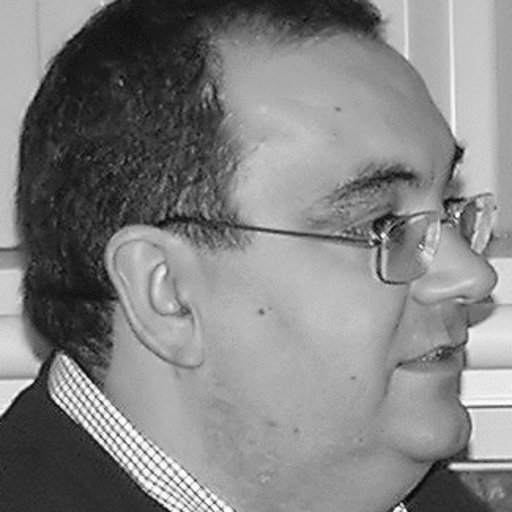### Semigroups of composition operators on Hardy spaces of Dirichlet series

Parole chiave

Abstract Let $\mathbb{C}_+ = \{ w\in \mathbb{C} : \text{Re}(w) > 0\}$ be the right half-plane. We consider continuous semigroups of holomorphic functions $\{ \Phi_t \}_{t \geq 0}$ in the so-called Gordon-Hedenmalm class $\mathcal{G}$, that is, the family of holomorphic functions $\Phi:\mathbb{C}_{+} \to \mathbb{C}_{+}$ giving rise to bounded composition operators in the Hardy space of Dirichlet series $\mathcal{H}^2$. We show that there is a one-to-one correspondence between continuous semigroups $\{\Phi_t\}_{t\geq0}$ in the class $\mathcal{G}$ and strongly continuous semigroups of composition operators $\{T_t\}_{t\geq0}$, where $T_t(f)=f\circ\Phi_t$, $f\in\mathcal{H}^2$. We characterize the infinitesimal generators of continuous semigroups in the class $\mathcal {G}$ as those Dirichlet series sending $\mathbb{C}_{+}$ into its closure. As a byproduct, and using results related to harmonic measures, we show that the functions $\Phi_{t}$ converge to the identity in the right half-plane.

We extend these results for the range $p\in[1,\infty)$. For the case $p=\infty$, we prove that there is no non-trivial strongly continuous semigroup of composition operators in $\mathcal{H}^\infty$. Some dynamical properties of these semigroups are obtained from a description of the Koenigs map of the semigroup.

This is a joint work with Carlos Gómez-Cabello and Luis Rodríguez-Piazza.

© 2021 GAA@polimi Generato con Hugo e inspirato al tema Resume Ultimo aggiornamento: 20 Marzo 2023